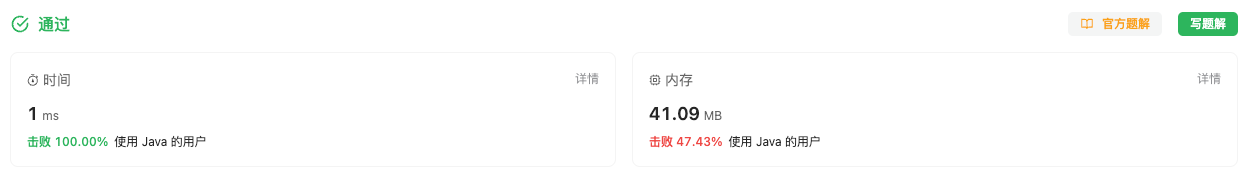#### 2586. 统计范围内的元音字符串数

###### 来源: 每日一题 2023.11.07

``````输入：words = ["are","amy","u"], left = 0, right = 2

- "are" 是一个元音字符串，因为它以 'a' 开头并以 'e' 结尾。
- "amy" 不是元音字符串，因为它没有以元音字母结尾。
- "u" 是一个元音字符串，因为它以 'u' 开头并以 'u' 结尾。

``````

``````输入：words = ["hey","aeo","mu","ooo","artro"], left = 1, right = 4

- "aeo" 是一个元音字符串，因为它以 'a' 开头并以 'o' 结尾。
- "mu" 不是元音字符串，因为它没有以元音字母开头。
- "ooo" 是一个元音字符串，因为它以 'o' 开头并以 'o' 结尾。
- "artro" 是一个元音字符串，因为它以 'a' 开头并以 'o' 结尾。

``````

• `1 <= words.length <= 1000`
• `1 <= words[i].length <= 10`
• `words[i]` 仅由小写英文字母组成
• `0 <= left <= right < words.length`
``````class Solution {
public int vowelStrings(String[] words, int left, int right) {

}
}
``````

#### 分析与题解

• 暴力模拟法

这个题这么简单我都能做错.... 一开始我认为 `left``right` 说的是每一个单词的情况, 也就是每一个单词的情况从 `[left, right]` 中判断, 但我看到的测试用例不都这个范围吗? 所以我就认为 `[left, right]` 区间没有啥用.

结果, `[left, right]` 区间说的是整个words数组的情况.

那么就简单了, 我们创建一个方法来判断某个字母是否是元音字母.

``````public boolean isVowel(char ch) {
return ch == 'a' || ch == 'e' || ch == 'i' || ch == 'o' || ch == 'u';
}
``````

然后再根据 `[left, right]` 的区间范围遍历 `words` 数组, 找出符合题意的单词即可.

``````for(int i = left; i <= right; i++) {
String word = words[i];
if(isVowel(word.charAt(0)) && isVowel(word.charAt(word.length() - 1))) {
result++;
}
}
``````

那么接下来, 我们就看一下整体的题解过程.

``````class Solution {
public int vowelStrings(String[] words, int left, int right) {
int result = 0;
for(int i = left; i <= right; i++) {
String word = words[i];
if(isVowel(word.charAt(0)) && isVowel(word.charAt(word.length() - 1))) {
result++;
}
}
return result;
}
public boolean isVowel(char ch) {
return ch == 'a' || ch == 'e' || ch == 'i' || ch == 'o' || ch == 'u';
}
}
``````

复杂度分析:

• 时间复杂度: O(n), 与字符串数组长度相关的时间复杂度.
• 空间复杂度: O(1), 常量基本的时间复杂度.

结果如下所示.IT界无底坑洞栋主 欢迎加Q骚扰:676758285